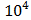# If the magnetic field of a plane electromagnetic wave is given by (The speed of light =3×/m/s) B=100×then the maximum electric field associated with it is (a) 4 ×N/C (b) 4.5 ×N/C (c) 6 ×N/C (d) 3 ×N/C

## Question ID - 50228 :- If the magnetic field of a plane electromagnetic wave is given by (The speed of light =3×/m/s) B=100×then the maximum electric field associated with it is (a) 4 ×N/C (b) 4.5 ×N/C (c) 6 ×N/C (d) 3 ×N/C

3537=100×=3×N/Ccorrect answer is 3×N/C

Next Question :
 A charge Q is disturbed over three concentric spherical shells of radii a, b, c (asuch that their surface charge densities are equal to one another. The total potential at a point at distance r from their common centre, where ra, would be : (a)(b)(c)(d)